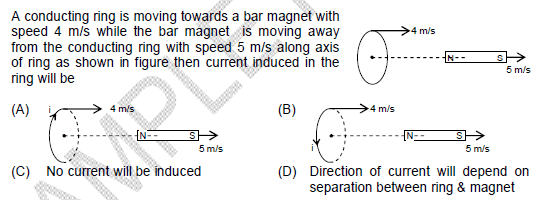# Direction of induced current in conducting ring due to motion of bar m

In summary, the question is about finding the direction of induced current in a conducting ring due to the motion of a bar magnet. After researching online, it was determined that the answer should be (B) based on the "reverse pole" rule and Lenz's law. However, there seems to be some confusion about the poles of the magnet and how they are counted, leading to the incorrect answer of (A). The explanation for the correct answer can be found in the provided web link.

## Homework Statement-

## The Attempt at a Solution

The relative velocity of magnet with respect to ring is 5−4=1m/s
How can I find the Direction of induced current in conducting ring due to motion of bar magnet?
I searched online and found this : http://www.phys.ufl.edu/courses/phy2049/f07/lectures/2049_ch30B.pdf

Please help. Is there any rule for this ? ( Like the right hand thumb rule for induced magnetic field ?)

When one tries to find the direction of induced current, the poles of the magnets also count. In the question the south pole goes first, and its description is given under the sub-heading 'Reverse pole' in web link you gave...
And yes, there is a law for this one and it is called the 'Lenzs law'.
The below one is a wikipedia link for the law..
http://en.wikipedia.org/wiki/Lenz's_law
Regards

Abhilash H N said:
When one tries to find the direction of induced current, the poles of the magnets also count. In the question the south pole goes first, and its description is given under the sub-heading 'Reverse pole' in web link you gave...
And yes, there is a law for this one and it is called the 'Lenzs law'.
The below one is a wikipedia link for the law..
http://en.wikipedia.org/wiki/Lenz's_law
Regards
I don't understand. Using the reverse pole rule on that webpage I'm getting the answer as (A) , can you please explain why the answer is (B)?
Edit: Got it.

Last edited: